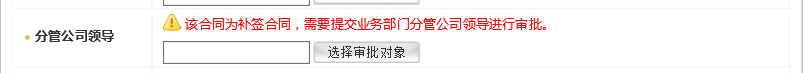JQuery写判断语句问题 本人菜鸟小白
`````` <tr id="rmbAmountFlag" style="display:none;">
<th width="18%">主管一级领导</th>
<td colspan="3">
<span class="twarning"><font color="red">按照公司规定，一般合同不需公司领导审批，若确需公司领导审批，请在此选择；否则此处请留空。</font></span>
<br>
<input type="hidden" name="partManageUser" id="partManageUser"/>
<input type="button" id="button" class="botton01" value="选择审批对象" onclick="openTreeOwner('partManageName','partManageUser','\${path}',1,'200000000',1,1)" />
</td>
</tr>
``````

`````` <tr id="YQManager" style="display:none;">
<th width="18%">分管公司领导</th>
<td colspan="3">
<span class="twarning"><font color="red">该合同为补签合同，需要提交业务部门分管公司领导进行审批。</font></span>
<br>
<input type="hidden" name="yqManageUser" id="yqManageUser"/>
<input type="button" id="button" class="botton01" value="选择审批对象" onclick="openTreeOwner('yqManageName','yqManageUser','\${path}',1,'200000000',1,1)" />
</td>
</tr>
``````根据判断语句选择页面选项是否显示，

（如果YQManager=为补签合同 且 rmbAmountFlag=大于两百万的合同 则隐藏补签领导选择页面选项 .ftl页面）

23个回答

``````
<div id="dv">页面选项内容.....</div>
<script>
var a = 2, b = 3;
if(a==2&&b==3)document.getElementById('dv').style.display='none'
</script>
``````2 年多之前 回复dong_wa qq_38793572 2018.04.13 15:52 if(a == 2 && b == 3){ \$("页面选项").hide(); }
2 年多之前 回复qq_32106973 兄弟可以帮我详细看一下吗 页面代码我上传了
2 年多之前 回复

if(a == 2 && b == 3){
\$("#tab").hide(); //tab为页面选项的id，可修改，不可重复
}

if(a == 2 && b == 3){
\$("页面选项").hide();
}

if(a=="2"&& b=="3"){
\$("#id").hide();//id为选项ID
}

/c:if
``````                <c:if test="\${type == 1}">
</c:if>
</td>

这是我写的一个demo里面用到的，判断type==0或者type==1的时候显示想要展示的内容。
``````

if (Number(a) == 2 && Number(a) == 3) {
}
Number，强制转换为数字格式

jquery选择器，\$("#选项id") \$("选项class")....还有多种选择方式

if(a == 2 && b == 3)

var a=2,b=3;
\$(function(){
if("#id").hide();
})

var a = 2, b = 3; if(a==2&&b==3)document.getElementById('dv').style.display='none'